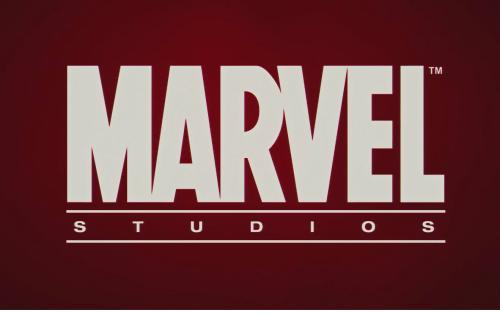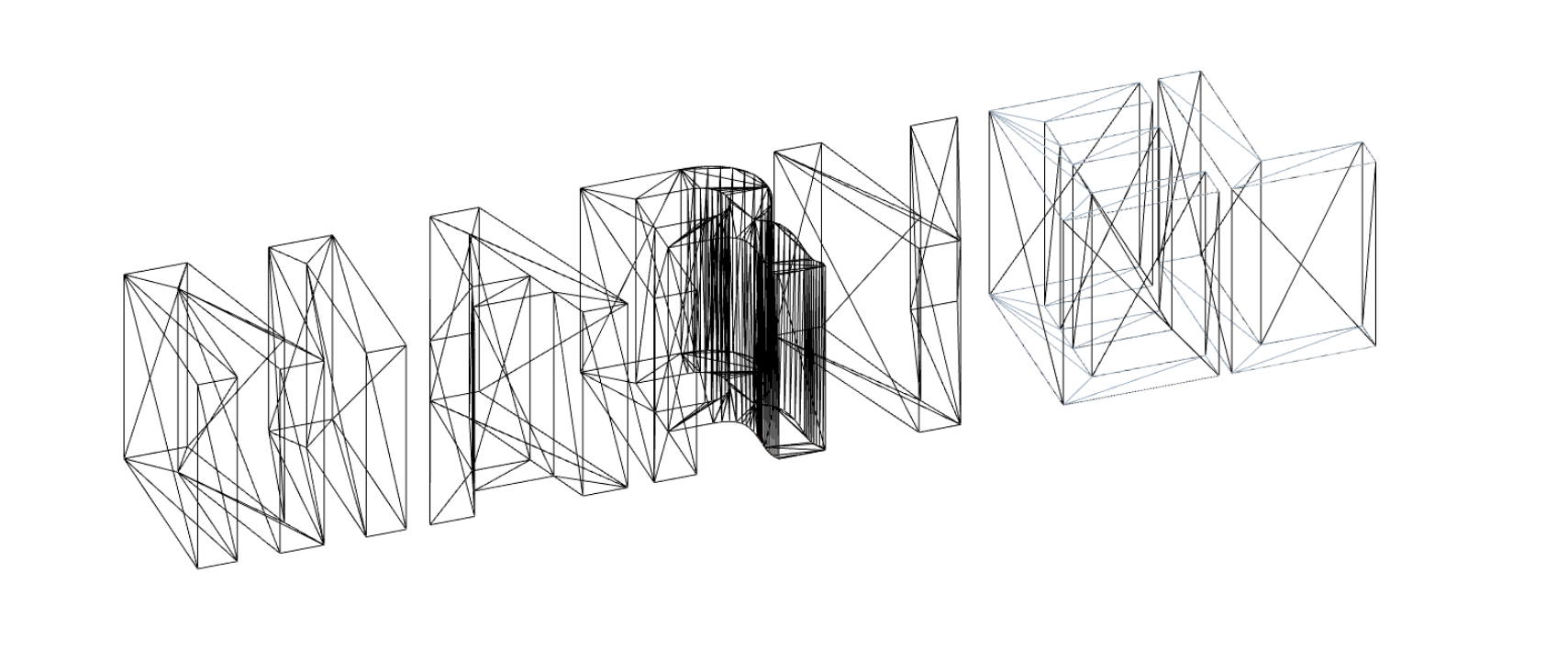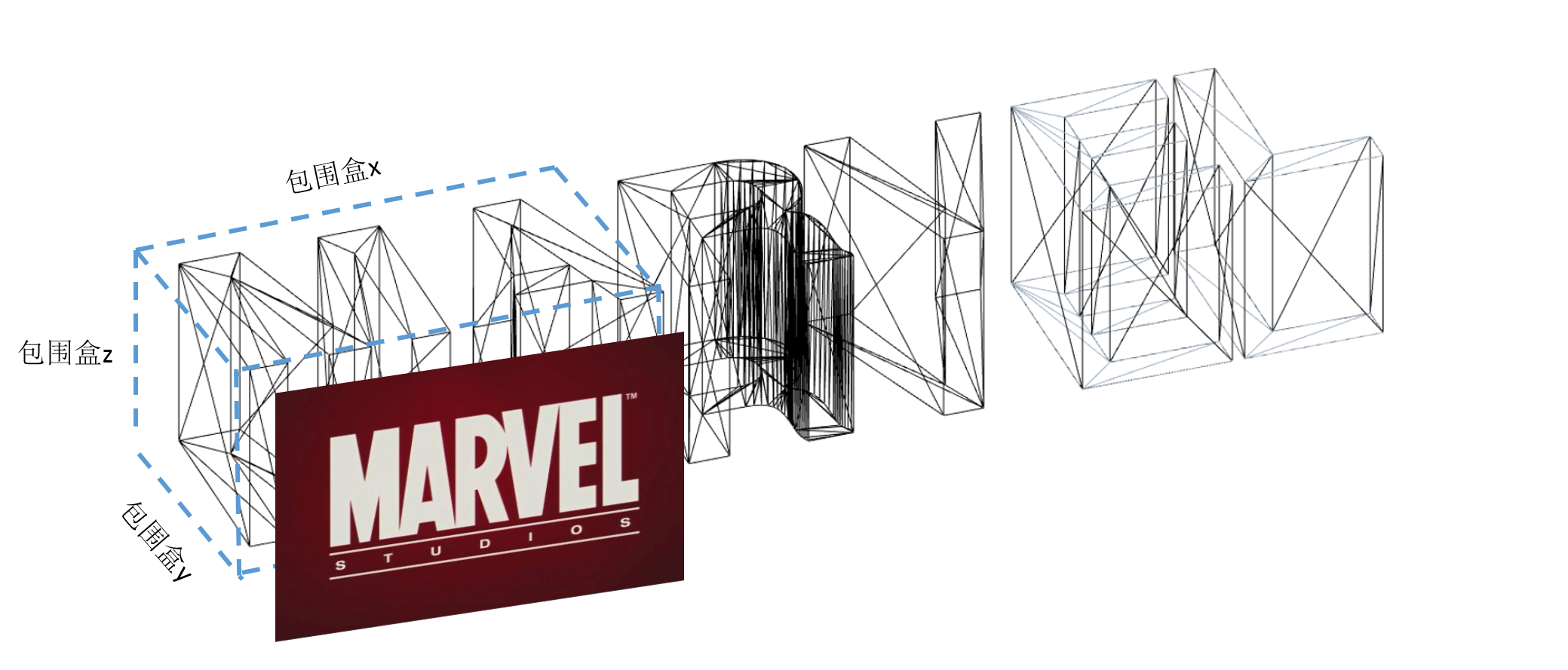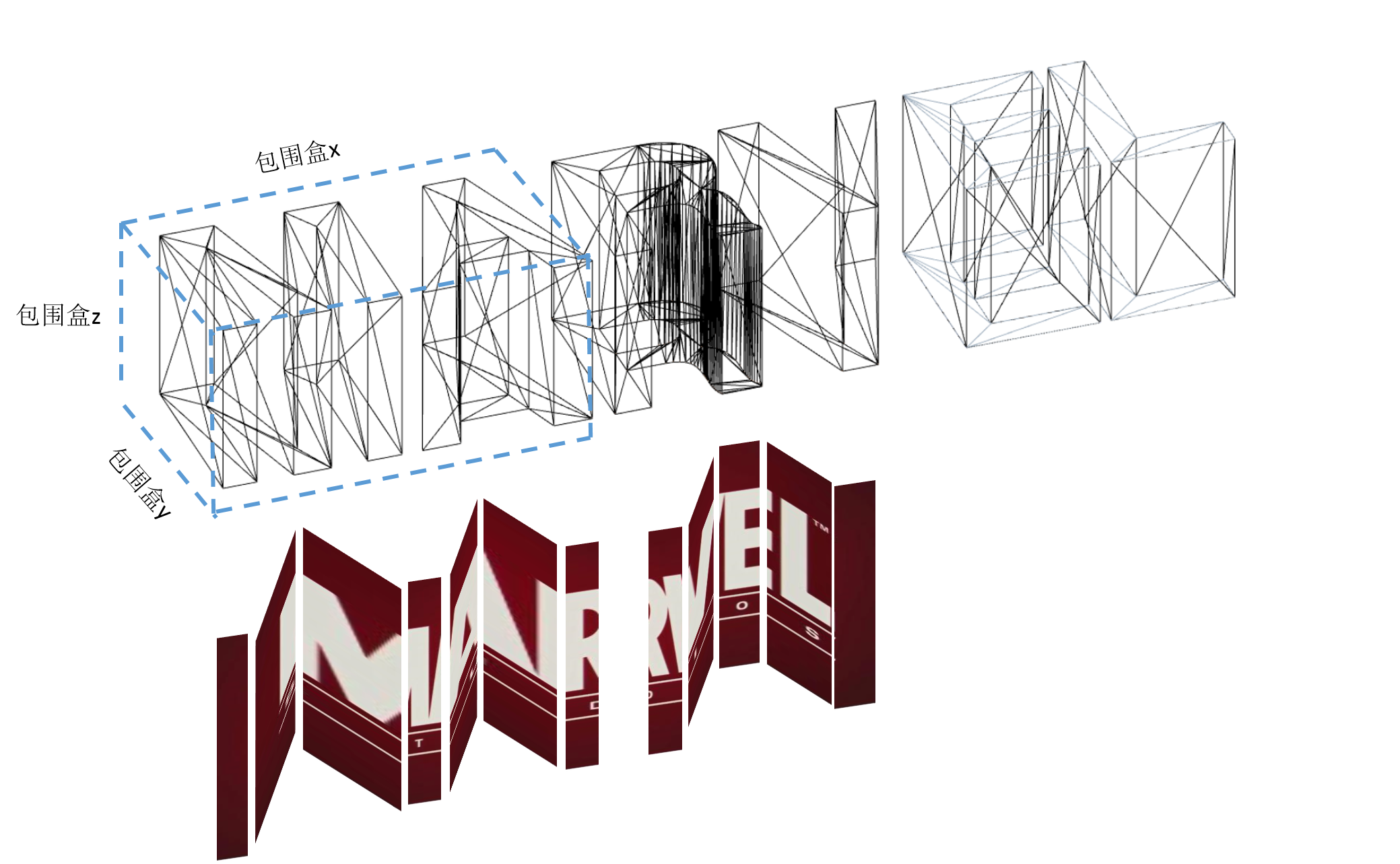# 【带着canvas去流浪（13）】用Three.js制作简易的MARVEL片头动画（下）## 一. 模型的制作

### 1.1 生成字体模型

var loader = new THREE.FontLoader();
var geometry = new THREE.TextGeometry( 'MARVEL',{
font: font,
size: 80,//平面形状大小
height: 5,//拉伸高度
curveSegments: 12,//默认使用的三角面片数，越多则表面越平滑
bevelEnabled: true,//是否使用倒角
bevelThickness: 10,
bevelSize: 8,
bevelOffset: 0,
bevelSegments: 5
} );
} );


//geometry指字体模型实例
geometry.computeBoundingBox();

//计算过一次以后，包围盒属性就会添加到geometry.boundingBox上，然后就可以以此为依据进行调整
cube.position.x = - (font.boundingBox.max.x - font.boundingBox.min.x)/2 + tempPos;
cube.position.y = - (font.boundingBox.max.y - font.boundingBox.min.y)/2;
cube.position.z = - (font.boundingBox.max.z - font.boundingBox.min.z)/2;### 1.2 多表面贴图A的字体模型内部有一个封闭空间，其中也有法向量z值小于0的面，它们也会按照同样的坐标转换标准被贴图。示例demo中的自动贴图相关代码如下：

//重新计算uv贴图部分
function rebuildUV(geo) {
if(!geo.isGeometry) return;

const max = geo.boundingBox.max;
const min = geo.boundingBox.min;
const offset = new THREE.Vector3(0 - min.x,0 - min.y, 0 - min.z);
const range = new THREE.Vector3(max.x  -  min.x,max.y - min.y, max.z - min.z);
const faces = geo.faces;

geo.faceVertexUvs  = [];

for(let i = 0; i< faces.length; i++){
const v1 = geo.vertices [faces [i] .a],
v2 = geo.vertices [faces [i] .b],
v3 = geo.vertices [faces [i] .c];

//faces[i].normal中为归一化的向量，可以表明面的指向
if(faces[i].normal.z > 0){
//z > 0 的面面向屏幕正面，与使用者相对。
geo.faceVertexUvs.push([
new THREE.Vector2((v1.x+offset.x)/range.x,(v1.y+offset.y)/range.y),
new THREE.Vector2((v2.x+offset.x)/range.x,(v2.y+offset.y)/range.y),
new THREE.Vector2((v3.x+offset.x)/range.x,(v3.y+offset.y)/range.y)
]);
}else if (faces[i].normal.y < 0){
// y < 0 的面为模型下面
geo.faceVertexUvs.push([
new THREE.Vector2((v1.x+offset.x)/range.x,(v1.z+offset.z)/range.z),
new THREE.Vector2((v2.x+offset.x)/range.x,(v2.z+offset.z)/range.z),
new THREE.Vector2((v3.x+offset.x)/range.x,(v3.z+offset.z)/range.z),
])
} else{
//没有用到的表面默认为不贴图
geo.faceVertexUvs.push([
new THREE.Vector2(0,0),
new THREE.Vector2(0,0),
new THREE.Vector2(0,0)
]);
}
}
geo.uvsNeedUpdate = true;
}


## 二. 镜头及动画

OrthographicCamera( left : Number, right : Number, top : Number, bottom : Number, near : Number, far : Number )
left — Camera frustum left plane.
right — Camera frustum right plane.
top — Camera frustum top plane.
bottom — Camera frustum bottom plane.
near — Camera frustum near plane.
far — Camera frustum far plane.


)

//模拟相机移动轨迹
function mockCameraTrack() {
//1.初始轨迹调整相机参数，将镜头拉远
if (camera.right < windowWidth) {

//镜头位置
camera.left -= step;
camera.right += step;
camera.top += step;
camera.bottom -= step;
}

//2.到达预设时间时开始反转
if (group.rotation.x < 0){
if(windowWidth - camera.right < 200){
step = 3;
rotationStep = 0.6;
}
group.position.x += 0.2 * step;
group.rotation.x += rotationStep * Math.PI / 180;
group.rotation.z -= (rotationStep * 5 / 9) * Math.PI / 180;
}

//更新相机投影坐标
camera.updateProjectionMatrix();
}


## 三. 大作业总结

posted @ 2019-07-23 20:47  大史不说话  阅读(452)  评论(0编辑  收藏